# TSA Mean VI

LabVIEW 2014 Advanced Signal Processing Toolkit Help

Edition Date: June 2014

Part Number: 372656C-01

»View Product InfoDownload Help (Windows Only)

Owning Palette: Statistical Analysis VIs

Requires: Advanced Signal Processing Toolkit

Computes the mean values of a univariate or multivariate (vector) time series. Wire data to the Xt input to determine the polymorphic instance to use or manually select the instance.

Use the pull-down menu to select an instance of this VI.

 Select an instance TSA Mean (Waveform)TSA Mean (Array)TSA Vector Mean (Waveform)TSA Vector Mean (Array)

## TSA Mean (Waveform)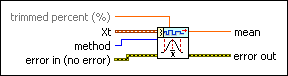trimmed percent specifies a percentage at which to discard the lowest and highest values in the time series. This option is available only when method is Trimmed.Xt specifies the univariate time series.method specifies the method to use in computing the mean value of the input time series. Refer to the Details section for more information about each method.

 0 Arithmetic (default) 1 Geometric 2 Trimmed 3 Medianerror in describes error conditions that occur before this node runs. This input provides standard error in functionality.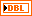mean returns the mean value of the input time series.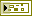error out contains error information. This output provides standard error out functionality.

## TSA Mean (Array)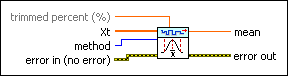trimmed percent specifies a percentage at which to discard the lowest and highest values in the time series. This option is available only when method is Trimmed.Xt specifies the univariate time series.method specifies the method to use in computing the mean value of the input time series. Refer to the Details section for more information about each method.

 0 Arithmetic (default) 1 Geometric 2 Trimmed 3 Medianerror in describes error conditions that occur before this node runs. This input provides standard error in functionality.mean returns the mean value of the input time series.error out contains error information. This output provides standard error out functionality.

## TSA Vector Mean (Waveform)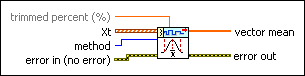trimmed percent specifies a percentage at which to discard the lowest and highest values in the time series. This option is available only when method is Trimmed.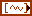Xt specifies the multivariate (vector) time series.method specifies the method to use in computing the mean value of the input time series. Refer to the Details section for more information about each method.

 0 Arithmetic (default) 1 Geometric 2 Trimmed 3 Medianerror in describes error conditions that occur before this node runs. This input provides standard error in functionality.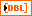vector mean returns the vector mean values of the multivariate (vector) time series.error out contains error information. This output provides standard error out functionality.

## TSA Vector Mean (Array)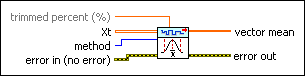trimmed percent specifies a percentage at which to discard the lowest and highest values in the time series. This option is available only when method is Trimmed.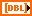Xt specifies the multivariate (vector) time series. Each column of the 2D array represents a vector at certain time.method specifies the method to use in computing the mean value of the input time series. Refer to the Details section for more information about each method.

 0 Arithmetic (default) 1 Geometric 2 Trimmed 3 Medianerror in describes error conditions that occur before this node runs. This input provides standard error in functionality.vector mean returns the vector mean values of the multivariate (vector) time series.error out contains error information. This output provides standard error out functionality.

## TSA Mean Details

This VI calculates the mean values of an input time series with the method you specify for the method parameter.

### Arithmetic mean

This VI calculates the arithmetic mean value according to the following equation: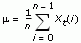where m is the arithmetic mean value, and n is the number of samples of the time series Xt.

### Geometric mean

This VI calculates the geometric mean value according to the following equation: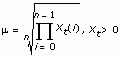where m is the geometric mean value, and n is the number of samples of the time series Xt.

### Trimmed mean

This VI calculates the trimmed mean value by eliminating a percentage of the smallest and largest values from the time series and then calculating the arithmetic average of the remaining values. This method is useful for a time series with extreme values.

### Median mean

This VI calculates the median mean value by sorting the values and then selecting the value in the middle. If the number of values in the sample is even, then the median is the average of the two middle values. This method generates a rough mean value but is useful in cases when a time series has large outliers.

## Example

Refer to the Series Statistical Analysis VI in the labview\examples\Time Series Analysis\TSAGettingStarted directory for an example of using the TSA Mean VI.

Not Helpful